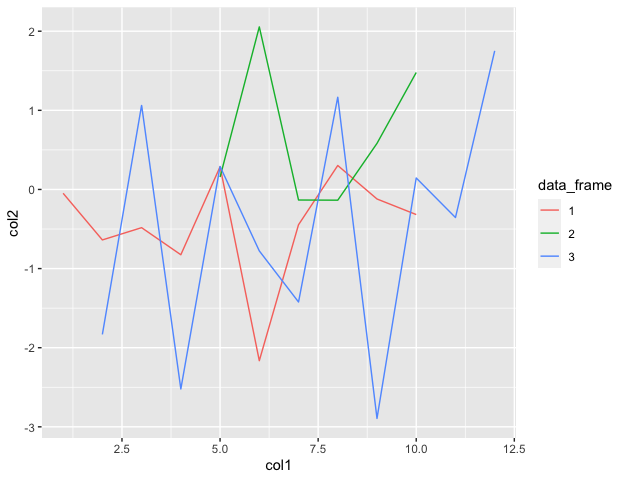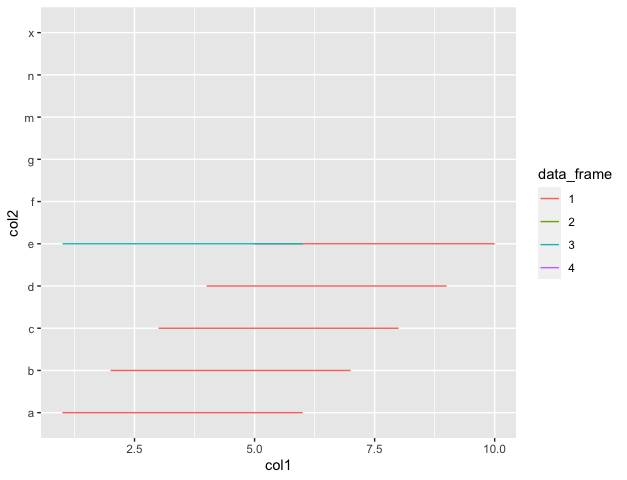Open in App
Not now

# Plot lines from a list of dataframes using ggplot2 in R

• Last Updated : 05 Nov, 2021

In this article, we will discuss how to plotlines in ggplot from a list of dataframes in the R programming language.

The ggplot2 package is used to visualize and analyze the data. The package can be downloaded and installed using the following command in R :

`install.packages("ggplot2")`

The ggplot method in R is used to do graph visualizations using the specified data frame. It is used to instantiate a ggplot object. Aesthetic mappings can be created to the plot object to determine the relationship between the x and y axis respectively. Additional components can be added to the created ggplot object.

Syntax: ggplot(data = NULL, mapping = aes(), colour())

Arguments :

• data – Default dataset to use for plot.
• mapping – List of aesthetic mappings to use for plot.

Geoms can be added to the plot using various methods. The geom_line() method in R can be used to add graphical lines in the plots made. It is added as a component to the existing plot. Aesthetic mappings can also contain color attributes that are assigned differently based on different dataframes. The geom_line() method has the following syntax :

`geom_line()`

Example 1:

## R

 `# importing required libraries``library``(``"dplyr"``)``library``(``"ggplot2"``)`` ` ` ` `# creating the dataframes``df1 = ``data.frame``(col1=``c``(1: 10), col2=``rnorm``(10))``df2 = ``data.frame``(col1=``c``(5: 10), col2=``rnorm``(6))``df3 = ``data.frame``(col1=``c``(2: 12), col2=``rnorm``(11))`` ` `# creating a list of dataframes``samplelist = ``list``(df1, df2, df3)``# plotting the data``graph < - ``ggplot``(``bind_rows``(samplelist, .id=``"data_frame"``), ``                 ``aes``(col1, col2, colour=data_frame)) +``geom_line``()`` ` `# printing the graph``print``(graph)`

Output

``` "First Dataframe"
> print(df1)
col1       col2
1     1  2.6799001
2     2  1.6732359
3     3 -0.2821830
4     4  0.6951255
5     5  0.3629730
6     6  1.6543411
7     7  0.9301622
8     8  0.6858366
9     9  1.3150289
10   10 -0.9306804
> print("Second Dataframe")
 "Second Dataframe"
> print(df2)
col1       col2
1    5 -0.1813050
2    6  1.3543525
3    7  0.0810269
4    8  0.1788353
5    9  1.5264921
6   10  0.3677910
> print("Third Dataframe")
 "Third Dataframe"
> print(df3)
col1       col2
1     2 -1.0602057
2     3 -0.6040208
3     4  1.9346507
4     5  0.5183120
5     6  0.7176499
6     7  0.2908290
7     8  1.4760342
8     9  0.5935123
9    10  0.3882407
10   11  0.8871490
11   12 -0.3974801```Example 2:

## R

 `# importing required libraries``library``(``"dplyr"``)``library``(``"ggplot2"``)`` ` ` ` `# creating the dataframes``df1 = ``data.frame``(col1=``c``(1: 10), col2=``letters``[1:5])``df2 = ``data.frame``(col1=``c``(7: 9), col2=``letters``[5:7])``df3 = ``data.frame``(col1=``c``(1: 6), col2=``rep``(``'e'``, 6))``df4 = ``data.frame``(col1=``c``(5, 9, 10), col2=``c``(``'x'``, ``'m'``, ``'n'``))`` ` `print``(``"First DataFrame"``)``print``(df1)`` ` `print``(``"Second DataFrame"``)``print``(df2)`` ` `print``(``"Third DataFrame"``)``print``(df3)`` ` `print``(``"Fourth DataFrame"``)``print``(df4)`` ` `# creating a list of dataframes``samplelist = ``list``(df1, df2, df3, df4)`` ` `# plotting the data``graph < - ``ggplot``(``bind_rows``(samplelist, .id=``"data_frame"``), ``                 ``aes``(col1, col2, colour=data_frame)) +``geom_line``()`` ` `# printing the graph``print``(graph)`

Output

``` "First DataFrame"
> print(df1)
col1 col2
1     1    a
2     2    b
3     3    c
4     4    d
5     5    e
6     6    a
7     7    b
8     8    c
9     9    d
10   10    e
> print ("Second DataFrame")
 "Second DataFrame"
> print(df2)
col1 col2
1    7    e
2    8    f
3    9    g
> print ("Third DataFrame")
 "Third DataFrame"
> print(df3)
col1 col2
1    1    e
2    2    e
3    3    e
4    4    e
5    5    e
6    6    e
> print ("Fourth DataFrame")
 "Fourth DataFrame"
> print(df4)
col1 col2
1    5    x
2    9    m
3   10    n```My Personal Notes arrow_drop_up